##### Geometry For DummiesWhen two lines intersect to make an X, angles on opposite sides of the X are called vertical angles. These angles are equal, and here’s the official theorem that tells you so.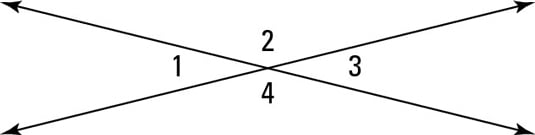Vertical angles are congruent: If two angles are vertical angles, then they’re congruent (see the above figure).

Vertical angles are one of the most frequently used things in proofs and other types of geometry problems, and they’re one of the easiest things to spot in a diagram. Don’t neglect to check for them!

Here’s an algebraic geometry problem that illustrates this simple concept: Determine the measure of the six angles in the following figure.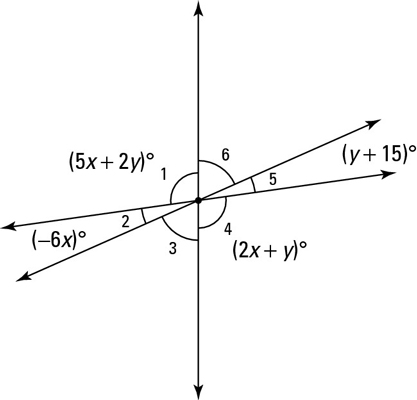Vertical angles are congruent, so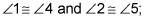and thus you can set their measures equal to each other: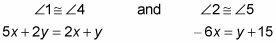Now you have a system of two equations and two unknowns. To solve the system, first solve each equation for y:

y = –3x

y = –6x – 15

Next, because both equations are solved for y, you can set the two x-expressions equal to each other and solve for x:

–3x = –6x – 15

3x = –15

x = –5

To get y, plug in –5 for x in the first simplified equation:

y = –3x

y = –3(–5)

y = 15

Now plug –5 and 15 into the angle expressions to get four of the six angles: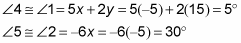To get angle 3, note that angles 1, 2, and 3 make a straight line, so they must sum to 180°: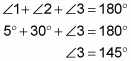Finally, angle 3 and angle 6 are congruent vertical angles, so angle 6 must be 145° as well. Did you notice that the angles in the figure are absurdly out of scale? Don’t forget that you can’t assume anything about the relative sizes of angles or segments in a diagram.